+

# FIFO

##### Rating:
(1)
• (0)
• (0)
• (0)
• (0)
• (1)
Author: Sophia Tutorial
##### Description:

Calculate the ending inventory and cost of goods sold using the FIFO method.

(more)
Tutorial
what's covered
This tutorial will cover the inventory cost flow assumption known as FIFO, which is an acronym for First In First Out.

Our discussion breaks down as follows:

1. FIFO
2. FIFO and Cost of Goods Sold

## 1. FIFO

FIFO is an inventory valuation method, which stands for First In First Out:As an inventory valuation method, FIFO helps to provide information about cost of goods sold and ending inventory. Under FIFO, goods that were purchased first are the first to be sold, meaning goods are assumed to be sold oldest to newest. A benefit to using FIFO is that it resembles the physical flow of goods.

EXAMPLE

Grocery stores or electronic stores, for example, want the inventory that they purchased first--their oldest products--to be sold first. In the case of grocery stores, they want to sell the oldest merchandise first so that their food doesn't spoil.

big idea
FIFO is First In First Out. Goods are assumed to be sold oldest to newest, which means that the most recent purchases are what remain in the ending inventory.

## 2. FIFO and Cost of Goods Sold

Next we will discuss FIFO and cost of goods sold. Remember, the cost of goods sold calculation starts with beginning inventory, then we add cost of goods purchased, which gives us goods available for sale. Then, we subtract out ending inventory, which equals cost of goods sold.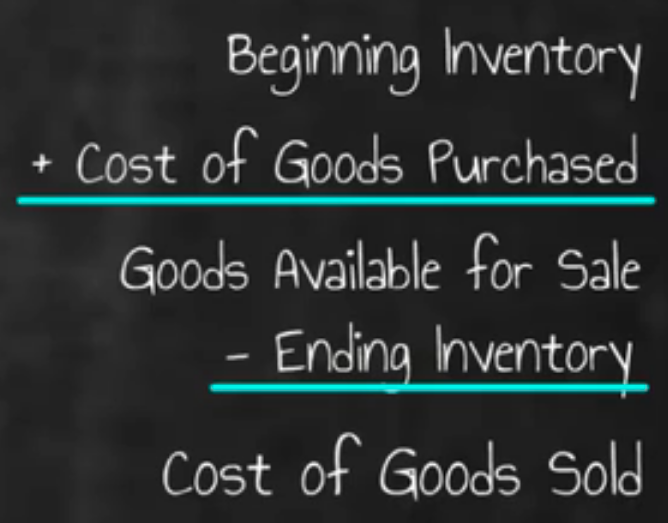Let's walk through an example of calculating cost of goods sold using the inventory valuation method of FIFO. Below you will see a spreadsheet outlining the cost of goods sold calculation. We will begin with the first line, beginning inventory. Beginning inventory is pulled from our balance sheet or from our trial balance. You can also see there is a schedule, detailing beginning inventory and purchases.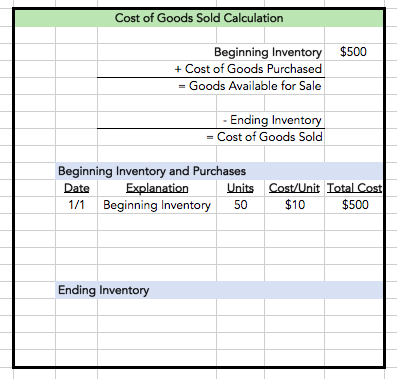Once we have plugged in beginning inventory, we need to determine cost of goods purchased. If you look at the detail of all the purchases made, you can see that we made three purchases throughout the year.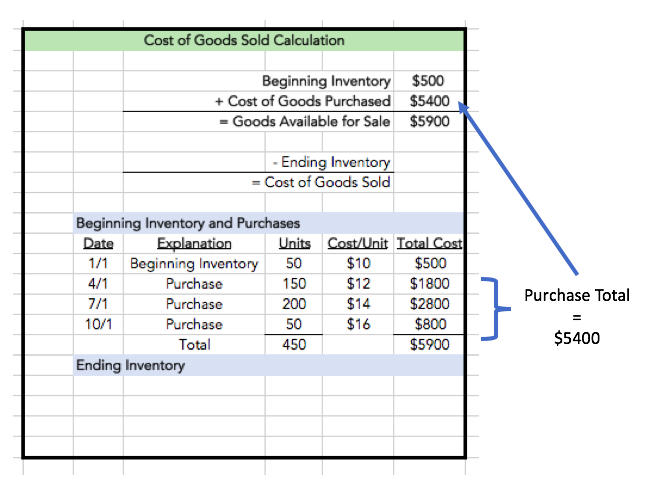We can take the total of those purchases, which is \$5,400, and drop that into the schedule, which means we now have total goods available for sale of \$5,900. Note that this is the total of beginning inventory plus all of the purchases.

Now we need to know what our ending inventory is. If you look at the ending inventory schedule below, we're going to make the assumption that we have 100 units left in our ending inventory.

So, if we're using FIFO and we have 100 units left, what does that mean? Which units are left in inventory? Under FIFO--First In First Out--, the oldest units (the units that came in first) were the first units to be sold. So when we are looking at ending inventory, we will be left with the newest units as our inventory. Start with the most recent purchase and work our way back.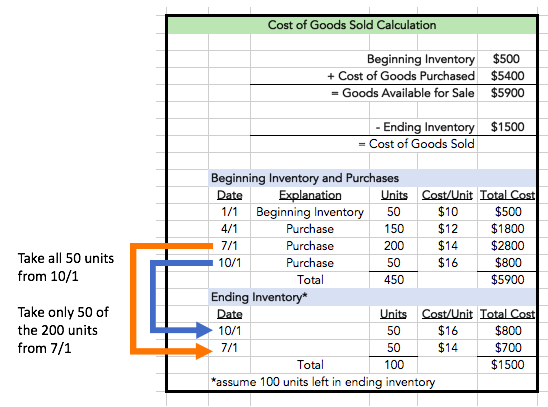If we have 100 units left, we know that we can take 50 units from the purchase made on the most recent purchase of 10/1, and then we can take another 50 units from the purchase made on July 1. This means that our ending inventory, 100 units, is \$1,500.

Then, we take that number, and drop it into our cost of goods sold calculation. We subtract out our ending inventory to give us cost of goods sold.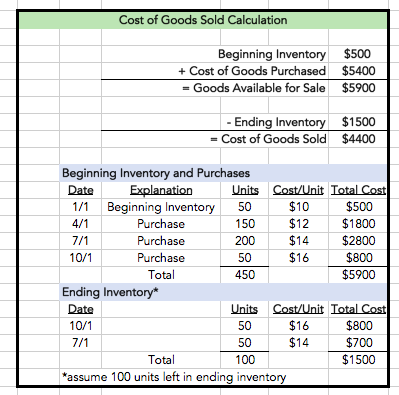In summary, to perform our cost of goods sold calculation, we started with our beginning inventory and added cost of goods purchased, to give us goods available for sale. We subtracted out ending inventory, which we calculated using FIFO, meaning the most recent purchases are the units that are assumed to be remaining in inventory.

summary
Today we learned about FIFO, First In First Out, which is an inventory valuation method that helps to provide information about cost of goods sold as well as ending inventory. Under FIFO, goods are assumed to be sold oldest to newest, so the oldest inventory items are sold first and the newer inventory items are what remains in ending inventory. We also performed a calculation of cost of goods sold using FIFO.

Source: Adapted from Sophia instructor Evan McLaughlin.

Rating Header